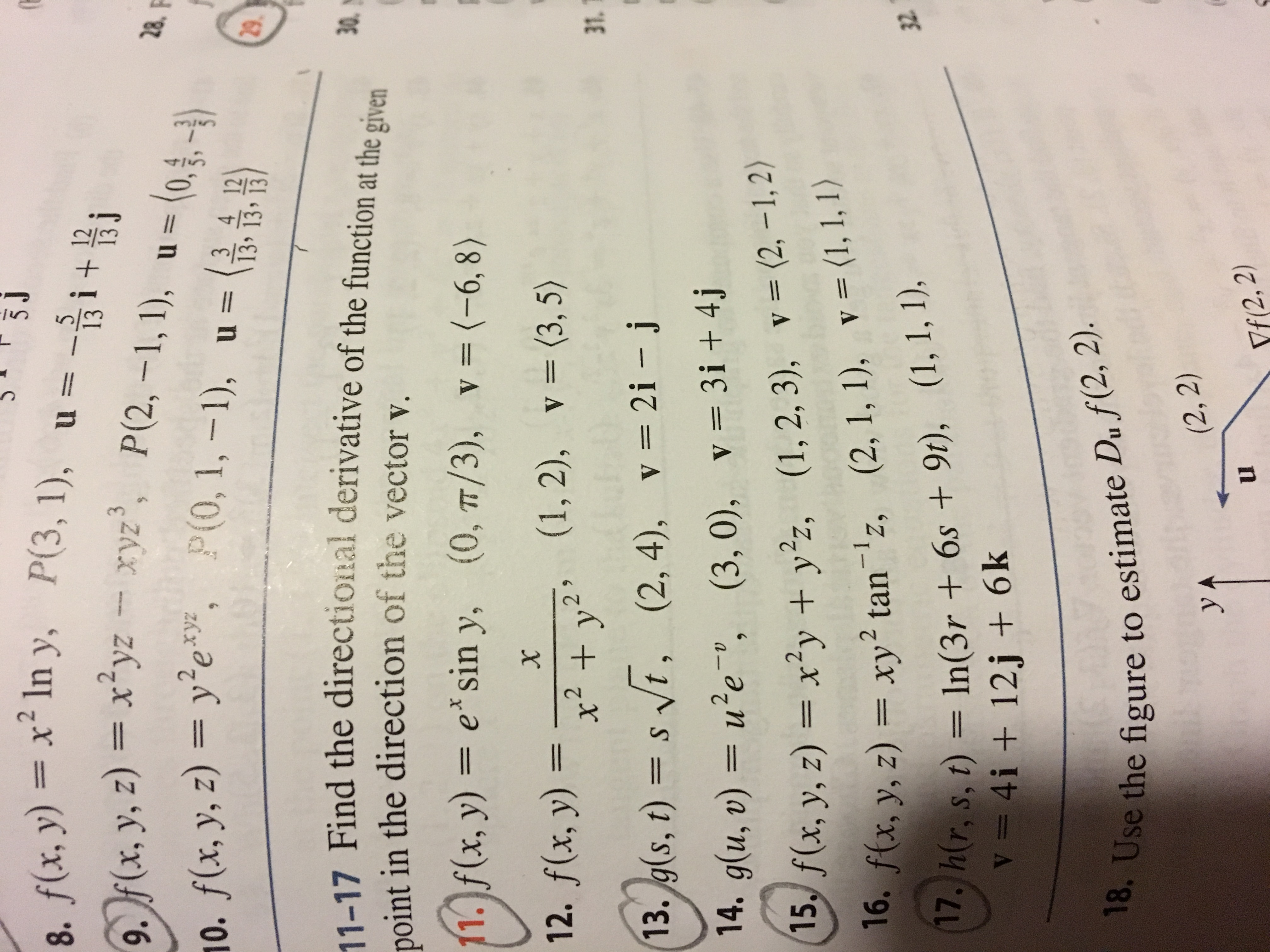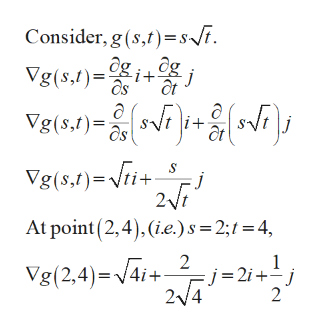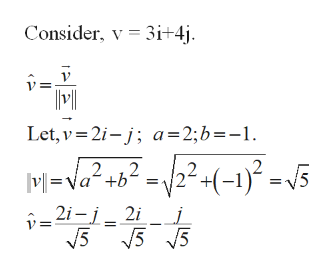# 3J8. f(x, y)xIn y, P(3, 1), u =513f(x, y, z) xyzxyz, P(2, -1, 1),28.(0,,)110. f(x, y, z) ye, (0, 1,-1), u=3 4 1213י1342911-17 Find the directional derivative of the function at the given0in the direction of the vector vpoint(0, TT/3), v= (-6, 8)11. f(x, y) = e*sin y,(0, T/3), v = (-6, 8)(1, 2), v (3, 5)12. f(x, y) =x22 *+ y31(2,4), v 2i -j13. g(s, t) = s t,(3, 0), v= 3i + 4j14. g(u, v) = ue,v= (2, -1,2)f(x, y, z) = x2y + yz,16. f(x,(1, 2, 3),v= (1, 1, 1)(2, 1, 1),y, z) = xy2 tan'z,17. h(r, s, t) In(3r + 6s +9t), (1, 1, 1),V= 4i + 12j + 6k3218. Use the figure to estimate Du f(2, 2).(2, 2)yAYf(2, 2)

Question
15 views

#13help_outlineImage Transcriptionclose3J 8. f(x, y)xIn y, P(3, 1), u = 5 13 f(x, y, z) xyzxyz, P(2, -1, 1), 28. (0,,) 11 0. f(x, y, z) ye, (0, 1,-1), u= 3 4 12 13י134 29 11-17 Find the directional derivative of the function at the given 0 in the direction of the vector v point (0, TT/3), v= (-6, 8) 11. f(x, y) = e*sin y, (0, T/3), v = (-6, 8) (1, 2), v (3, 5) 12. f(x, y) = x2 2 * + y 31 (2,4), v 2i -j 13. g(s, t) = s t, (3, 0), v= 3i + 4j 14. g(u, v) = ue, v= (2, -1,2) f(x, y, z) = x2y + yz, 16. f(x, (1, 2, 3), v= (1, 1, 1) (2, 1, 1), y, z) = xy2 tan'z, 17. h(r, s, t) In(3r + 6s +9t), (1, 1, 1), V= 4i + 12j + 6k 32 18. Use the figure to estimate Du f(2, 2). (2, 2) yA Yf(2, 2) fullscreen
check_circle

Step 1

Find directional derivative of function in the direction v.

Step 2

Find the gradient of function g at the point (2, 4).help_outlineImage Transcriptionclosei Consider, g (s,f)s Ve0.4)- Vg(s,f) Vg(s,t) ct as Vg(s,f)ri+ j 2 At point (2,4), (i.e.) s = 2; 1 = 4, Vg(2,4)4i+ 2_j=21 +1 2/4 2 fullscreen
Step 3

Find a unit vector...help_outlineImage TranscriptioncloseConsider, v 3i+4j Let, v 2i-; a=2;b=-1 M-VO P2(-1)-5 |v=a+b' 2i-j V5 2i 5 5 fullscreen

### Want to see the full answer?

See Solution

#### Want to see this answer and more?

Solutions are written by subject experts who are available 24/7. Questions are typically answered within 1 hour.*

See Solution
*Response times may vary by subject and question.
Tagged in

### Other